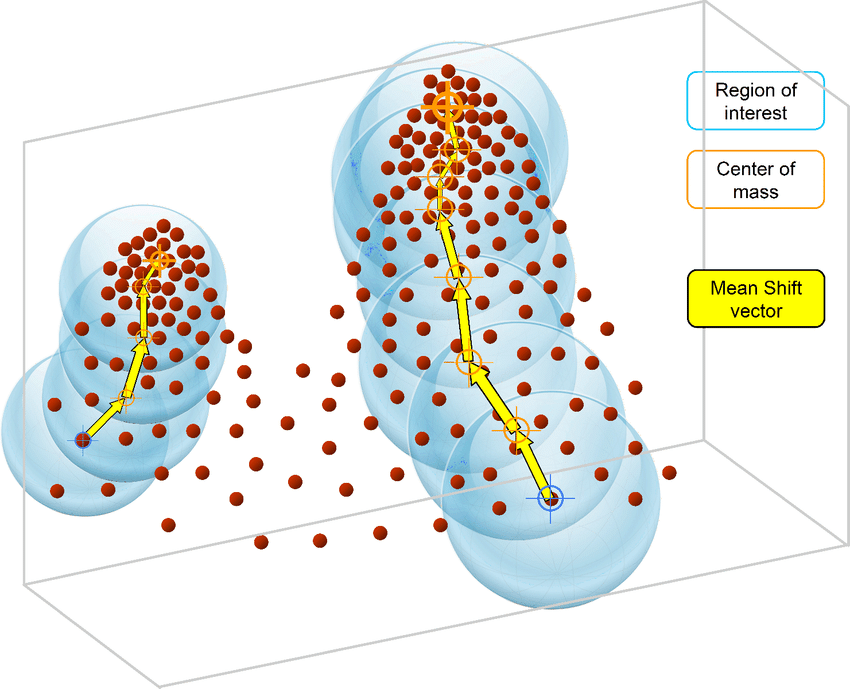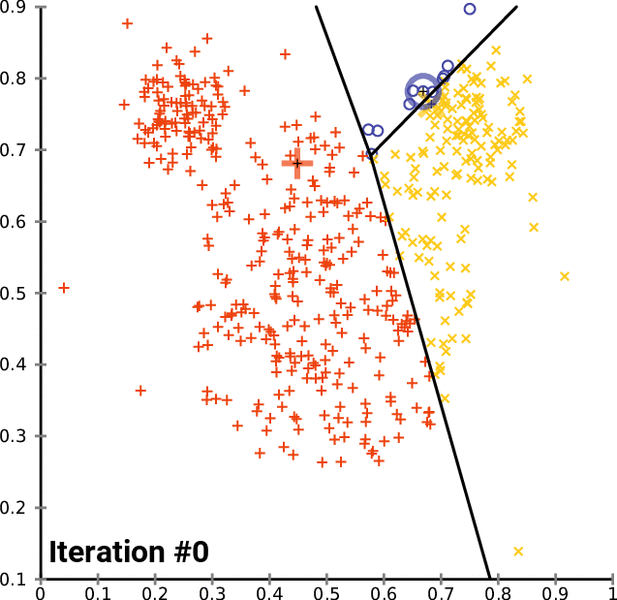## How to program the Mean Shift algorithm

Mean Shift is an unsupervised machine learning algorithm. It is a hierarchical data clustering algorithm that finds the number of clusters a feature space should be divided into, as well as the location of the clusters and their centers. It works by grouping data points according to a “bandwidth”, a distance around data points, and converging the clusters’ centers towards the densest regions of data.Continue reading “How to program the Mean Shift algorithm”

## How to program the K-Means clustering algorithm

K-Means is a popular unsupervised machine learning algorithm for data clustering. A typical start for flat clustering, the K-Means algorithm works by defining a number K of clusters to be extracted by the algorithm. With this K number given, the algorithm will then find the best “centroids” to cluster the data around.Continue reading “How to program the K-Means clustering algorithm”